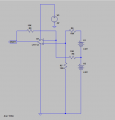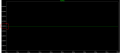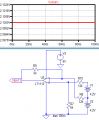# Battery differential Voltage measurement help needed

#### mishra87

Joined Jan 17, 2016
975

#### mishra87

Joined Jan 17, 2016
975
Could any body help me out to calculate the theoretical ?

#### sghioto

Joined Dec 31, 2017
3,715
Probably homework but OK.
Assuming input impedance of ADC is infinite and LR voltage is correct.
Voltage at input of ADC is:

Case 1....( 8.4 X 20K)/(20K+22K) -.7

Case 2.....(4.2 X 20K)/(20K+22K) + 4.2 -.7

Case 3.....(4.2 X 20K)/(20K+22K) -.7
Steve G

#### mishra87

Joined Jan 17, 2016
975
Probably homework but OK.
Assuming input impedance of ADC is infinite and LR voltage is correct.
Voltage at input of ADC is:

Case 1....( 8.4 X 20K)/(20K+22K) -.7

Case 2.....(4.2 X 20K)/(20K+22K) + 4.2 -.7

Case 3.....(4.2 X 20K)/(20K+22K) -.7
Steve G
Many Thanks !
It is not homework.

#### mishra87

Joined Jan 17, 2016
975
Hi Friend,

I wanted to measure battery voltage of upper cell with 4V opamp supply.
here is the file i tried with simulation but i could not see the vout as expected.

Since opamp supply is 4V i have lower down battery voltage at input Side.

Could anyone explain me how it can be done ?#### Attachments

• 3 KB Views: 2

#### sghioto

Joined Dec 31, 2017
3,715
Could anyone explain me how it can be done ?
You need to increase the supply voltage to the op amp.
SG

#### mishra87

Joined Jan 17, 2016
975
You need to increase the supply voltage to the op amp.
SG
No Supply voltage is fixed, it can not increase !
I want reduce the voltage at input side itself with some scaling factor !

#### sghioto

Joined Dec 31, 2017
3,715
The LT1112 is not rail to rail, max output with 4 volt supply is around 3.75v depending on the load.
What output are you expecting to read with the circuit as designed?
SG

#### mishra87

Joined Jan 17, 2016
975
The LT1112 is not rail to rail, max output with 4 volt supply is around 3.75v depending on the load.
What output are you expecting to read with the circuit as designed?
SG
Thanks you so much for interest and reply !

I just wanted to read the voltage of upper cell i.e. lets sat 4.2V of fully charged Li Ion battery !
I just took random opamp from LT spice.
My supply voltage is fixed i.e. 4V.
Now i wanted scale down the battery voltage at input side let say if vbat = 4.2V, opamp output is 3.6V or something like. Since i know the scaling factor i can add in calculation.

Hope now you have perception in your mind !
Regards,

#### mishra87

Joined Jan 17, 2016
975
Hi,

You may use LT1494 rail to rail opamp.

Regards,

#### mishra87

Joined Jan 17, 2016
975
Use a difference amp. The reading is the difference between the supply (V1 + V2) and V2 which = V1. You don't need a separate 5 volt supply.
SGView attachment 215209

Still you did not get my points what I was trying to say !

I wanted to operate opamp with microcontroller supply voltage which is 4V. I do not want give opamp supply of 8.4V I.e battery voltage total !

#### Danko

Joined Nov 22, 2017
1,492
Now i wanted scale down the battery voltage at input side let say if vbat = 4.2V, opamp output is 3.6V or something like. Since i know the scaling factor i can add in calculation.
I wanted to operate opamp with microcontroller supply voltage which is 4V. I do not want give opamp supply of 8.4V I.e battery voltage total !
Do you want something like this:R9/R8=R7/R10=SCALE
(V1+V2)/V3 should be < than (R7+R10)/R7

Last edited:

#### mishra87

Joined Jan 17, 2016
975
Do you want something like this:
View attachment 215222

Yes i wanted to something like this !
Simulation looks very good but you explain a bit in theory how did it 2.1V ?
I wanted to understand concept for measurement.

Thanks once again !

#### sghioto

Joined Dec 31, 2017
3,715

Still you did not get my points what I was trying to say !

I wanted to operate opamp with microcontroller supply voltage which is 4V. I do not want give opamp supply of 8.4V I.e battery voltage total !
Is this just an exercise like homework or a real circuit you are planning on using? Why measure battery voltage then have to add a "scaling" factor to get the value when you can get the reading directly as I suggested.

SG

#### ci139

Joined Jul 11, 2016
1,873
R9/R8=R7/R10=SCALE
(V1+V2)/V3 should be < than (R7+R10)/R7
Differential Amplifier Configuration
Figure 7 shows a differential amplifier configured for offset adjustment. ...
// the Vos in above figure (at the link) is the merging point of the Rf , the R1 and the R2
// the Vos can be set by multiple means
// the formula defines your Vo
// if your Rf = Ri then the voltage gain would be 1
// if your Rf = N·Ri then the voltage gain would be N
// the amplified input voltage N · Vin is simply shifted by the offset voltage Vos
// in the other words the output voltage Vo is referenced to Vos
// the (output voltage shift) Vos = SGND (Signal Ground) which is also the Vss (the Op Amp's negative rail voltage) ... in your case ... is ±0 (Zero) V
// e.g.-- your output signal has no offset respective to the potentials at the SGND and at the Vss

#### mishra87

Joined Jan 17, 2016
975
Last edited:

#### ci139

Joined Jul 11, 2016
1,873
200µA ($$\rightarrow\ I_C≈40mA$$) is likely enough to fully turn on the BJT (unless it has to transmit a highr power)
you are driving the Q5(NPN) with 3.3mA ... you may do so ... but depending on how fast you need it to be switched - you might save some power there

if your output is the battery voltage reading ... then you might want to use constant current sinks instead of the Q3 Q5 ... otherwise you have the Vce drop (that may be varying) affecting your output :: about https://wiki.analog.com/university/courses/electronics/text/chapter-11 see 11.6 Basic MOSFET current mirror Figure ( Fig. 11.7 Simple MOS current mirror ) -- you may use the BJT current mirror but it is a bit more tricky to tune and it's also less stable = more dependent on the supply voltage

Last edited: